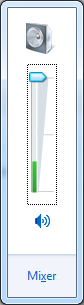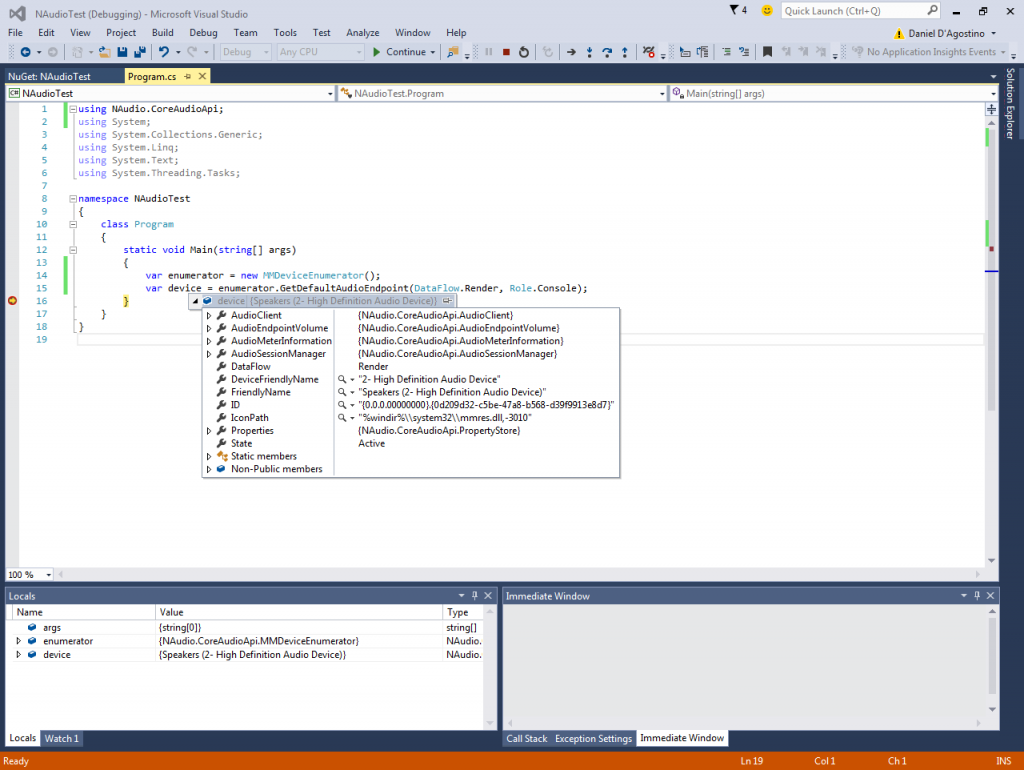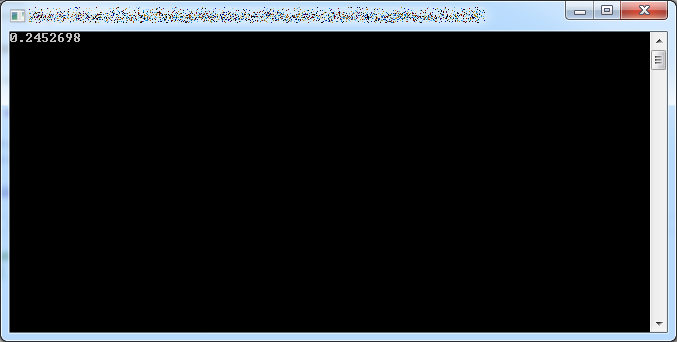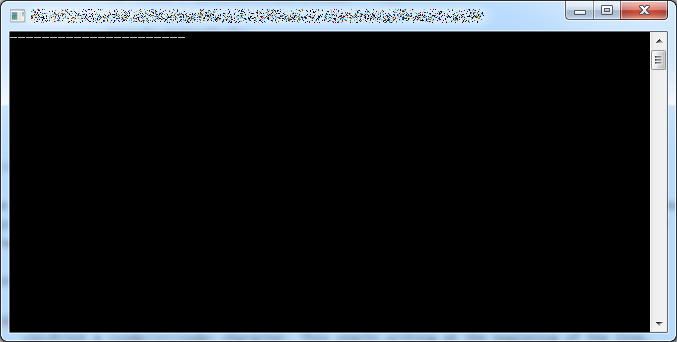Displaying a Volume Meter using NAudio

Windows 7 has this volume meter in the system tray. I’m sure you’re pretty familiar with it.It’s pretty cool. If you’re playing music, the green bar dances up and down, reflecting the volume of the music being played at the time.

If you’re developing an application that includes some form of multimedia, such as the ability to play music or listen to recorded phone calls, something simple like this is a great way to provide feedback to the user, showing that something is actually playing.

Typically, doing anything with sound in Windows is a messy business, as you’d have to play around with some pretty low-level APIs.To get an idea, just take a look at how many different ways there are to get master sound volume in C#.

Fortunately, there’s a managed library called NAudio which takes away a lot of the pain of dealing with this stuff. And quite conveniently, it’s available as a NuGet package.

After installing NAudio via NuGet, you can get a reference to your default audio output device using the following code:

var enumerator = new MMDeviceEnumerator();
var device = enumerator.GetDefaultAudioEndpoint(DataFlow.Render, Role.Console);

Note that there are three different values for Role, but they don’t seem to make any difference.

Put a breakpoint at the end and hover over the device variable to see that it’s bound to your actual output audio device:From this, we can get a value that indicates the audio volume:

while (true)
{
Console.Write("\r{0}", device.AudioMeterInformation.MasterPeakValue);
}

I’m using the old \r trick to overwrite the value displayed in the same line with each iteration. You’ll see that the volume displayed is a float value:That’s nice, but it’s a bit hard to visually interpret how it’s changing. Let’s build a simple volume meter in the console instead.

while (true)
{
var volume = device.AudioMeterInformation.MasterPeakValue;
var scale = (int)Math.Floor(volume * 79);

Console.Write("\r");

var sb = new StringBuilder();
sb.Append('-', scale);
sb.Append(' ', 79 - scale);
Console.Write(sb.ToString());
}

We can represent the volume meter as a series of dashes in a line in the command line. Since NAudio gives us a value between 0 and 1, we can scale that to something between 0 and 79 (since 80 characters fit in a line in the command line by default).

With that done, we can then:

1. Print a \r character. This starts writing at the beginning of the line, essentially overwriting the previously drawn meter.
2. Print a number of dashes proportional to the scaled volume we just calculated. Since we’re using StringBuilder’s repeat ability, we don’t need loops.
3. Print spaces for the rest of the line. This clears any leftover dashes from the previously drawn meter.

And there, you have it:This screenshot doesn’t really do it justice. Since the meter is updating pretty much in real time, go ahead and run this program when you have music playing to see how nicely it dances. 🙂

Calculating the Checksum of a FIX Message

The FIX (Financial Information Exchange) Protocol is an industry standard used by financial institutions to… guess what… exchange financial information.

A FIX message is simply a sequence of key-value pairs, each followed by a SOH (ASCII value 1) character. Since SOH is a non-printable character, FIX messages are often illustrated using another character, such as a pipe. The following is an example of a FIX message, taken from FIX Parser:

8=FIX.4.1|9=61|35=A|34=1|49=EXEC|52=20121105-23:24:06|56=BANZAI|98=0|108=30|10=003|

As you can see, key-value pairs are delimited by the pipe character (which would be a SOH character in a real FIX message). Note how there is also a pipe character at the very end. Keys are separated from values with an equals sign (=). The keys themselves are numbers; each number represents a particular field in the FIX protocol. For example, FIX Field 8 represents the FIX protocol as well as the beginning of a FIX message. The use of numbers instead of field names makes messages less readable, but keeps them small.

The last field in a FIX message is the checksum (FIX Field 10). The algorithm used to calculate the checksum is described here:

“The checksum of a FIX message is calculated by summing every byte of the message up to but not including the checksum field itself. This checksum is then transformed into a modulo 256 number for transmission and comparison. The checksum is calculated after all encryption is completed, i.e. the message as transmitted between parties is processed.

“For transmission, the checksum must be sent as printable characters, so the checksum is transformed into three ASCII digits.

“For example, if the checksum has been calculated to be 274 then the modulo 256 value is 18 (256 + 18 = 274). This value would be transmitted a |10=018| where “10=”is the tag for the checksum field.”

In C#, we can implement this as follows.

First, let’s create our test message. We start with a pipe-delimited representation (just because it’s more readable that way), without the checksum field, and then we swap out the pipes for SOH characters (\u0001). Since FIX uses an ASCII representation, we can easily get a byte representation of the message:

string message = "8=FIX.4.1|9=61|35=A|34=1|49=EXEC|52=20121105-23:24:06|"
+ "56=BANZAI|98=0|108=30|";
message = message.Replace('|', '\u0001');
byte[] messageBytes = Encoding.ASCII.GetBytes(message);

The algorithm itself is then easy to implement. We simply iterate over each byte, add its value to a running total, divide it by 256, and take the remainder:

for (int i = 0; i < message.Length; i++)
total += messageBytes[i];

int checksum = total % 256;

There’s nothing more to add – that’s the checksum. If you’re sending a FIX message, you’ll convert the value to a string and pad it with zeroes if necessary to make sure it’s exactly 3 characters:

string checksumStr = checksum.ToString().PadLeft(3, '0');

If you’re parsing a received message, you’ll want to compare the checksum you calculated to the one provided with the message, to make sure the message isn’t corrupt.